Updating search results...# Grade 5 DLC Blended Math

This collection features resources developed by The Distance Learning Centre (DLC) for blended math in Grade 5.

78 affiliated resources

# Search Resources

View
Selected filters: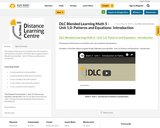Conditional Remix & Share Permitted
CC BY-NC-SA
Rating
0.0 stars

The purpose of this lesson is to introduce the unit on patterns and equations.

Included is a YouTube video to support Grade 5 Blended Learning Math - Unit 1.0: Patterns and Equations - Introduction.

Subject:
Math
Material Type:
Activity/Lab
Homework/Assignment
Lesson
Provider:
Sun West Distance Learning Centre (DLC)
12/05/2023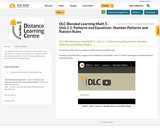Conditional Remix & Share Permitted
CC BY-NC-SA
Rating
0.0 stars

The purpose of this lesson is to explore number patterns and pattern rules.

Included is a YouTube video to support Grade 5 Blended Learning Math - Unit 1.1: Patterns and Equations- Number Patterns and Pattern Rules.

Subject:
Math
Material Type:
Activity/Lab
Homework/Assignment
Lesson
Provider:
Sun West Distance Learning Centre (DLC)
12/05/2023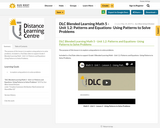Conditional Remix & Share Permitted
CC BY-NC-SA
Rating
0.0 stars

The purpose of this lesson is to explore using patterns to solve problems.

Included is a YouTube video to support Grade 5 Blended Learning Math - Unit 1.2: Patterns and Equations- Using Patterns to Solve Problems.

Subject:
Math
Material Type:
Activity/Lab
Homework/Assignment
Lesson
Provider:
Sun West Distance Learning Centre (DLC)
12/05/2023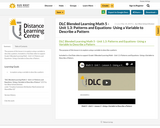Conditional Remix & Share Permitted
CC BY-NC-SA
Rating
0.0 stars

The purpose of this lesson is to explore using a variable to describe a pattern.

Included is a YouTube video to support Grade 5 Blended Learning Math - Unit 1.3: Patterns and Equations- Using a Variable to Describe a Pattern.

Subject:
Math
Material Type:
Activity/Lab
Homework/Assignment
Lesson
Provider:
Sun West Distance Learning Centre (DLC)
12/05/2023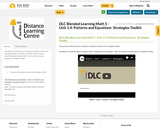Conditional Remix & Share Permitted
CC BY-NC-SA
Rating
0.0 stars

The purpose of this lesson is to explore strategies to add to the strategies toolkit.

Included is a YouTube video to support Grade 5 Blended Learning Math - Unit 1.4: Patterns and Equations- Strategies Toolkit.

Subject:
Math
Material Type:
Activity/Lab
Homework/Assignment
Lesson
Provider:
Sun West Distance Learning Centre (DLC)
12/05/2023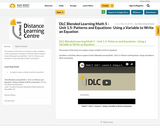Conditional Remix & Share Permitted
CC BY-NC-SA
Rating
0.0 stars

The purpose of this lesson is to explore using a variable to write an equations.

Included is a YouTube video to support Grade 5 Blended Learning Math - Unit 1.5: Patterns and Equations- Using a Variable to Write an Equation.

Subject:
Math
Material Type:
Activity/Lab
Homework/Assignment
Lesson
Provider:
Sun West Distance Learning Centre (DLC)
12/05/2023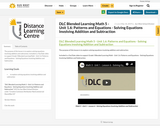Conditional Remix & Share Permitted
CC BY-NC-SA
Rating
0.0 stars

The purpose of this lesson is to explore solving equations involving addition and subtraction.

Included is a YouTube video to support Grade 5 Blended Learning Math - Unit 1.6: Patterns and Equations - Solving Equations Involving Addition and Subtraction.

Subject:
Math
Material Type:
Activity/Lab
Homework/Assignment
Lesson
Provider:
Sun West Distance Learning Centre (DLC)
12/05/2023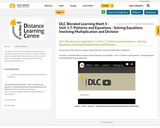Conditional Remix & Share Permitted
CC BY-NC-SA
Rating
0.0 stars

The purpose of this lesson is to explore solving equations involving multiplication and division.

Included is a YouTube video to support Grade 5 Blended Learning Math - Unit 1.7: Patterns and Equations - Solving Equations Involving Multiplication and Division.

Subject:
Math
Material Type:
Activity/Lab
Homework/Assignment
Lesson
Provider:
Sun West Distance Learning Centre (DLC)
12/05/2023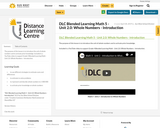Conditional Remix & Share Permitted
CC BY-NC-SA
Rating
0.0 stars

The purpose of this lesson is to introduce the unit of whole numbers and to activate prior knowledge.

Included is a YouTube video to support Grade 4 Blended Learning Math - Unit 2.0: Whole Numbers - Introduction.

Subject:
Math
Material Type:
Activity/Lab
Homework/Assignment
Lesson
Provider:
Sun West Distance Learning Centre (DLC)
12/05/2023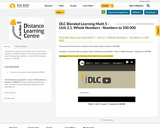Conditional Remix & Share Permitted
CC BY-NC-SA
Rating
0.0 stars

The purpose of this lesson is to explore and compare whole numbers to 100 000.

Included is a YouTube video to support Grade 5 Blended Learning Math - Unit 2.1: Whole Numbers - Numbers to 100 000.

Subject:
Math
Material Type:
Activity/Lab
Homework/Assignment
Lesson
Provider:
Sun West Distance Learning Centre (DLC)
12/05/2023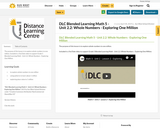Conditional Remix & Share Permitted
CC BY-NC-SA
Rating
0.0 stars

The purpose of this lesson is to explore whole numbers to one million.

Included is a YouTube video to support Grade 5 Blended Learning Math - Unit 2.2: Whole Numbers - Exploring One Million.

Subject:
Math
Material Type:
Activity/Lab
Homework/Assignment
Lesson
Provider:
Sun West Distance Learning Centre (DLC)
12/05/2023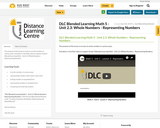Conditional Remix & Share Permitted
CC BY-NC-SA
Rating
0.0 stars

The purpose of this lesson is to learn to write numbers in various ways.

Included is a YouTube video to support Grade 5 Blended Learning Math - Unit 2.3: Whole Numbers - Representing Numbers.

Subject:
Math
Material Type:
Activity/Lab
Homework/Assignment
Lesson
Provider:
Sun West Distance Learning Centre (DLC)
12/05/2023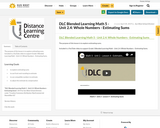Conditional Remix & Share Permitted
CC BY-NC-SA
Rating
0.0 stars

The purpose of this lesson is to explore estimating sums.

Included is a YouTube video to support Grade 5 Blended Learning Math - Unit 2.4: Whole Numbers - Estimating Sums.

Subject:
Math
Material Type:
Activity/Lab
Homework/Assignment
Lesson
Provider:
Sun West Distance Learning Centre (DLC)
12/05/2023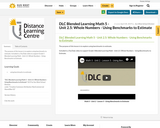Conditional Remix & Share Permitted
CC BY-NC-SA
Rating
0.0 stars

The purpose of this lesson is to explore using benchmarks to estimate.

Included is a YouTube video to support Grade 5 Blended Learning Math - Unit 2.5: Whole Numbers - Using Benchmarks to Estimate.

Subject:
Math
Material Type:
Activity/Lab
Homework/Assignment
Lesson
Provider:
Sun West Distance Learning Centre (DLC)
12/05/2023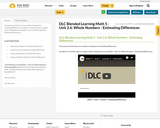Conditional Remix & Share Permitted
CC BY-NC-SA
Rating
0.0 stars

The purpose of this lesson is to explore strategies for estimating differences.

Included is a YouTube video to support Grade 5 Blended Learning Math - Unit 2.6: Whole Numbers - Estimating Differences.

Subject:
Math
Material Type:
Activity/Lab
Homework/Assignment
Lesson
Provider:
Sun West Distance Learning Centre (DLC)
12/05/2023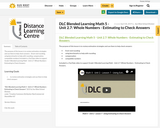Conditional Remix & Share Permitted
CC BY-NC-SA
Rating
0.0 stars

The purpose of this lesson is to review estimation strategies and use them to help check answers - (front-end rounding, using benchmarks to help with rounding, compensation, compatible numbers)

Included is a YouTube video to support Grade 5 Blended Learning Math - Unit 2.7: Whole Numbers - Estimating to Check Answers.

Subject:
Math
Material Type:
Activity/Lab
Homework/Assignment
Lesson
Provider:
Sun West Distance Learning Centre (DLC)
12/05/2023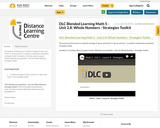Conditional Remix & Share Permitted
CC BY-NC-SA
Rating
0.0 stars

The purpose of this lesson is to add the strategy of "guess and check" or "guess and test" to a grade 5 mathematics estimation strategies toolkit.

Included is a YouTube video to support Grade 5 Blended Learning Math - Unit 2.8: Whole Numbers - Strategies Toolkit.

Subject:
Math
Material Type:
Activity/Lab
Homework/Assignment
Lesson
Provider:
Sun West Distance Learning Centre (DLC)
12/05/2023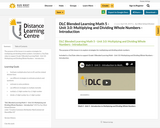Conditional Remix & Share Permitted
CC BY-NC-SA
Rating
0.0 stars

The purpose of this lesson is to explore strategies for multiplying and dividing whole numbers.

Included is a YouTube video to support Grade 5 Blended Learning Math - Unit 3.0: Multiplying and Dividing Whole Numbers - Introduction.

Subject:
Math
Material Type:
Activity/Lab
Homework/Assignment
Lesson
Provider:
Sun West Distance Learning Centre (DLC)
12/05/2023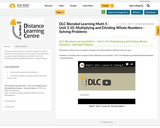Conditional Remix & Share Permitted
CC BY-NC-SA
Rating
0.0 stars

The purpose of this lesson is to explore strategies for solving problems with more than one step.

Included is a YouTube video to support Grade 5 Blended Learning Math - Unit 3.10: Multiplying and Dividing Whole Numbers - Solving Problems.

Subject:
Math
Material Type:
Activity/Lab
Homework/Assignment
Lesson
Provider:
Sun West Distance Learning Centre (DLC)
12/05/2023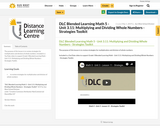Conditional Remix & Share Permitted
CC BY-NC-SA
Rating
0.0 stars

The purpose of this lesson is to review strategies for multiplication and division of whole numbers.

Included is a YouTube video to support Grade 5 Blended Learning Math - Unit 3.11: Multiplying and Dividing Whole Numbers - Strategies Toolkit.

Subject:
Math
Material Type:
Activity/Lab
Homework/Assignment
Lesson
Provider:
Sun West Distance Learning Centre (DLC)